# Feature AND

I'm going to take a break from looking at M&Ms this week and instead consider a question that Richard asked yesterday:

"I have two binary images of the same size. I want to select regions from image one, where that region has to partially overlap with a region from image two."

I've seen this called the "Feature-AND" operation, and you can use the Image Processing Toolbox function imreconstruct to do it. I'll use one of the toolbox sample images to show you how.

bw = imread('text.png');
imshow(bw)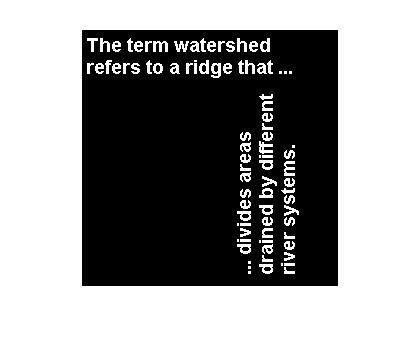Suppose I've got a "marker" image that has a thick line running down the diagonal, like this:

stripe = logical(eye(256));
stripe = imdilate(stripe, ones(3,3));
imshow(stripe)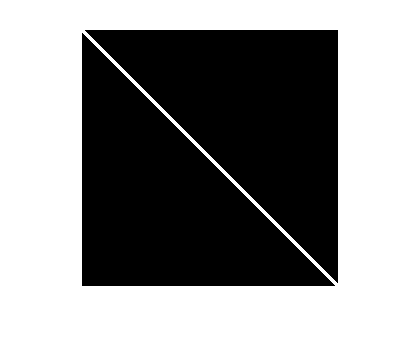Now ask the question, "Which objects in the original image touch the line?" It's easy to use a simple elementwise AND to see which pixels in the original image touch the line:

touching_pixels = bw & stripe;
imshow(touching_pixels)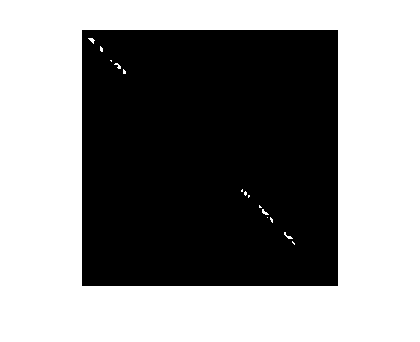Now to get the entire objects that are touching the line, apply an operation called morphological reconstruction, which is what imreconstruct does. This function takes two inputs, a marker image and a mask image. Use the touching pixels as the marker image, and use the original image as the mask image.

marker = touching_pixels;
imshow(out)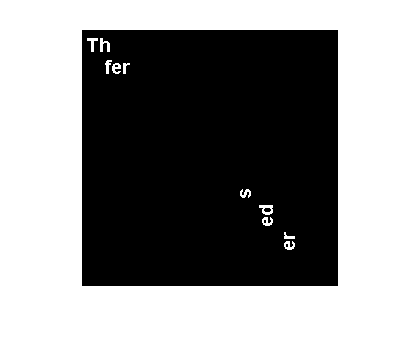Neat!

Here's another variation: "Which objects in the original image contain a vertical stroke that's at least 11 pixels tall?"

Start by eroding the original image by a vertical line structuring element.

se = strel(ones(11,1));
bw_eroded = imerode(bw, se);
imshow(bw_eroded)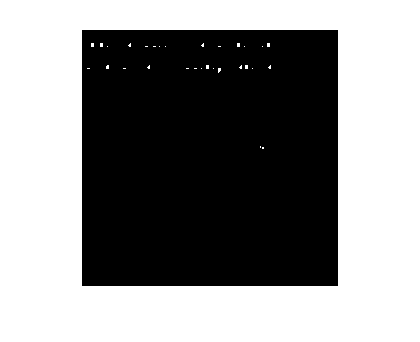You can see that many characters are gone completely. If we define the pixels that are left as the marker image, then reconstruction gives us back the complete objects:

marker = bw_eroded;
imshow(out)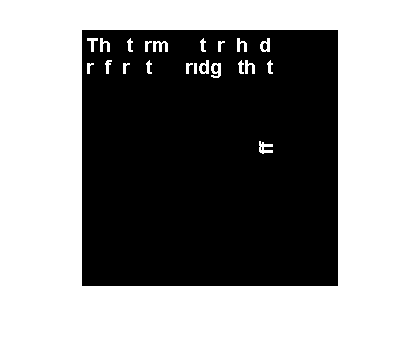This is a very useful operation, and I recommend that you add it to your bag of image processing tricks.

Published with MATLAB® 7.11

|